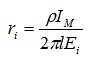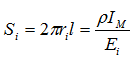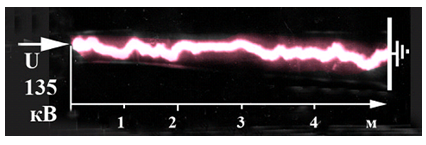# What you need to know about non-linear properties of soil

From the series of articles "Grounding in lightning protection - answers to frequently asked questions in the design".

It is necessary to repeat that soil is a very poor conductor. At the resistivity of about 100 Ohm m even quite a moderate current density σ = 104 А/m2 = 1 А/cm2 creates an electric field Egr = ρσ = 106VВ/m. It is enough for the start of ionization processes in the ground. The classical idea of the effects of ionization comes down to the fact that a well conducting plasma bag is formed at the surface of the grounding electrodes, which increases the area of electrode's contact with the ground. As a result, the grounding resistance of the electrode is reduced greater, the stronger the current is and thus the radius of the formed plasma is greater.

Elementary approximate estimation is useful here. For an extended electrode the length of which l is much greater than its radius r0, the grounding resistance is dependent on the geometric dimensions as R3 ~ l-1ln (l/r0). So reduction of grounding resistance with the increase of the radius due to plasma formation may not be very significant, because its value is under the logarithm sign. The radius of the ionized region ri can be estimated on the basis of the electric field intensity on its outer surface E(ri), which must be equal to the ionization threshold Ei. Then for the lightning current IM its density on the ionized surface σi = IM/ (2πril) will satisfy the condition σiρ = Ei. whereLet's make an assessment for a typical ground electrode system with three vertical electrodes 5 m in length and with a horizontal bus, prescribed by AD 34.21.122-87. Their total length is 25 m. In the ground with resistivity ρ = 100 Ohm * m at the lightning current of 100 kA, and Ei = 106 V / m, it gives ri ≈ 0,06 m instead of the typical metal electrode radius r0 ≈ 0.01 m. Substituting under logarithm shows that such an eightfold increase of the radius will reduce the resistance of a typical ground electrode by about 30%, which is not so critical. The effect is practically significant in high-resistivity ground only. For example, at ρ = 1000 Ohm * m ground ionization would lead to a reduction of earth resistance 2 times - although the result is quite significant, but still completely inadequate in order to equalize the resistance values of a typical ground electrode in soils with a resistivity of 100 and 1000 Ohm * m (remember that according to the point of view of AD 34.21.122-87 authors, a typical  ground electrode should be applied in any soil!).

In practical use, it is important to estimate the sizes of the grounding device, at which the ionization processes in the soil are able to somehow affect the value of the grounding resistance. A recently derived formula fits this target. According to it, the surface area supporting the ionization is determined asIn the ground of low resistance ρ = 1000 Ohm m at the lightning current IM = 100 kA the estimate gives the ultimate area of the surface ground electrodes of 10m2, At the radius of 10 mm their ultimate total length is defined as l <160 m. This means that the ionization processes cease to be relevant even in the assessment of the grounding contour, encircling the building 40x40 m in size. Only a pulse mode of rapidly rising current spreading in the time range of microseconds can make an exception, when a small fraction of grounding electrodes got involved into operation directly at the current injection  entrance point.

Lately, principally other consequences of ionization processes development in the soil attract attention. We are talking about moving spark channels. They can be formed from the insertion point of the lightning current into ground, extending along the ground surface. The mechanism of their development is fundamentally different from the mechanism of the formation of long sparks in the air. The main factor is the intense heating of the plasma channel by current leakage through the side surface contacting the ground. As a result, there is a voltage dramatic drop required for the formation of the spark channel.Figure 32

Photo in Fig. 34 reproduces the plasma channel along the ground surface  5 m long. For its formation in the laboratory, the voltage of 135 kV instead of 2500 kV necessary in a clean air environment, was required.

E. M. Bazelyan, DEA, professor
Energy Institute named after G.M. Krzyzanowski, Moscow